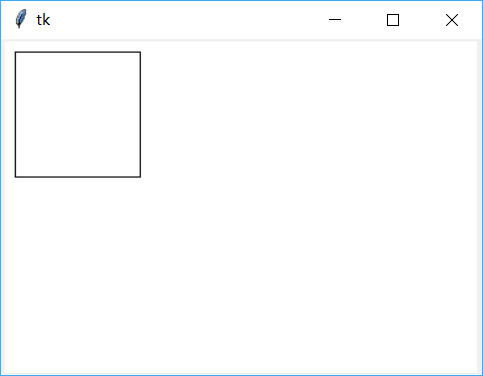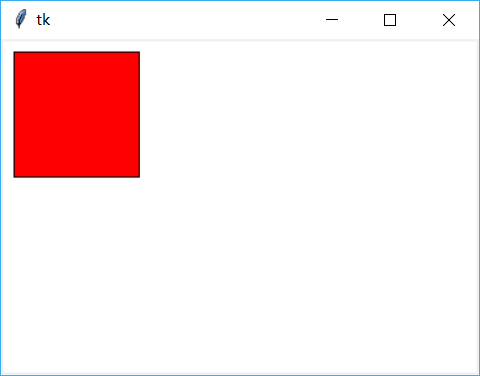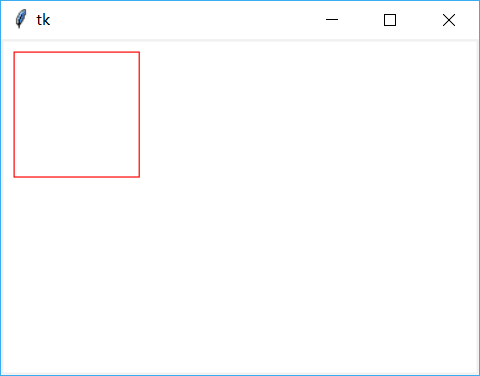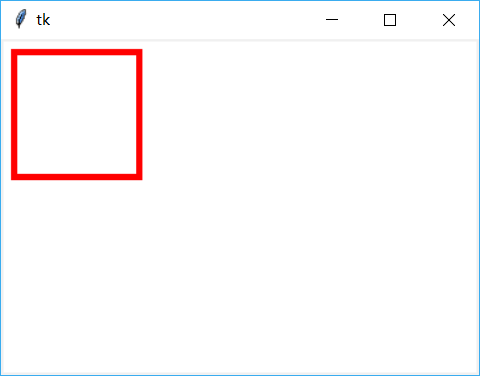## tkinter -- Canvas(1)

提供可以用来进行绘图的 Container，支持基本的几何元素，使用 Canvas 进行绘图时，所有的操作都是通过 Canvas，不是通过它的元素

元素的表示可以使用 handle 或 tag

第一个Canvas程序，指定画布的颜色为白色

代码:

```import tkinter as tk
root = tk.Tk()
# 创建一个 Canvas，设置其背景色为白色
cv = tk.Canvas(root, bg='white')
cv.pack()
root.mainloop()```

效果:为明显起见，将背景色设置为白色，用以区别 root

创建一个 item

创建一个矩形，指定画布的颜色为白色

代码:

```import tkinter as tk
root = tk.Tk()
# 创建一个 Canvas，设置其背景色为白色
cv = tk.Canvas(root, bg='white')
# 创建一个矩形，坐标为(10,10,110,110)
cv.create_rectangle(10, 10, 110, 110)
cv.pack()
root.mainloop()```

效果:为明显起见，将背景色设置为白色，用以区别 root

指定 item 的填充色

创建一个矩形，指定画布的背景色为白色

使用属性 fill 设置它的填充颜色

代码:

```import tkinter as tk
root = tk.Tk()
# 创建一个 Canvas，设置其背景色为白色
cv = tk.Canvas(root, bg='white')
# 创建一个矩形，坐标为(10,10,110,110)
# 指定矩形的填充色为红色
cv.create_rectangle(10, 10, 110, 110, fill='red')
cv.pack()
root.mainloop()```

效果:指定 item 的边框颜色

创建一个矩形，指定画布的背景色为白色

使用属性 outline 设置它的边框颜色

代码:

```import tkinter as tk
root = tk.Tk()
# 创建一个 Canvas，设置其背景色为白色
cv = tk.Canvas(root, bg='white')
# 创建一个矩形，坐标为(10,10,110,110)
cv.create_rectangle(10, 10, 110, 110, outline='red')
cv.pack()
root.mainloop()```

效果:指定边框的宽度

指定画布的背景色为白色

使用属性 width 指定线的宽度

代码:

```import tkinter as tk
root = tk.Tk()
# 创建一个 Canvas，设置其背景色为白色
cv = tk.Canvas(root, bg='white')
# 创建一个矩形，坐标为(10,10,110,110)
cv.create_rectangle(10, 10, 110, 110, outline='red', width=5)
cv.pack()
root.mainloop()```

结果:指定矩形的边框颜色为红色，设置线宽为5，注意与 Canvas 的 width 是不同的

画虚线

指定画布的背景色为白色

使用属性 dash,这个值只能为奇数

代码:

```import tkinter as tk
root = tk.Tk()
# 创建一个 Canvas，设置其背景色为白色
cv = tk.Canvas(root, bg='white')
# 创建一个矩形，坐标为(10,10,110,110)
cv.create_rectangle(10, 10, 110, 110, outline='red', dash=10, fill='yellow')
cv.pack()
root.mainloop()```

结果:指定矩形的边框颜色为红色,画虚线

使用画刷填充

指定画布的背景色为白色

使用属性 stipple

代码：

```import tkinter as tk
root = tk.Tk()
# 创建一个 Canvas，设置其背景色为白色
cv = tk.Canvas(root, bg='white')
# 创建一个矩形，坐标为(10,10,110,110)
cv.create_rectangle(10, 10, 110, 110, outline='red', stipple='gray12', fill='yellow')
cv.pack()
root.mainloop()```

效果:指定矩形的边框颜色为红色,自定义画刷

修改 item 的坐标

指定画布的背景色为白色

使用 Canvas 的方法来重新设置 item 的坐标

代码:

```import tkinter as tk
root = tk.Tk()
# 创建一个 Canvas，设置其背景色为白色
cv = tk.Canvas(root, bg='white')
# 创建一个矩形，坐标为(10,10,110,110)
rt = cv.create_rectangle(10, 10, 110, 110, outline='red', stipple='gray12', fill='yellow')
cv.pack()

# 重新设置rt的坐标(相当于移动一个item)
cv.coords(rt, (100, 100, 250, 250))
root.mainloop()```

结果:动态修改 item 的坐标